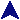Setting VariablesRuby Example Code# Class names must be capitalized.  Technically, it's a constant.
class Fred

# The initialize method is the constructor.  The @val is
# an object value.
def initialize(v)
@val = v
end

# Set it and get it.
def set(v)
@val = v
end

def to_s
return "Fred(val=" + @val.to_s + ")"
end

# Since a simple access function is so common, ruby lets you declare one
# automatically, like this:
# You can list any number of object variables. Separate by commas, and each
# needs its own colon
# attr_reader :fred, :joe, :alex, :sally
end

class Alice <Fred
# We have a message, too.
def initialize(n, m)
super(n)
@msg = m
end

# Takes the base result and changes the class name.
def to_s
ret = super
ret.gsub!(/Fred/, 'Alice')
return ret + ' ' + @msg + '!'
end

# The = allows the method to be used on the right, and the left of the
# assignment is the parameter.
def appmsg=(more)
@msg += more
end

# Like attr_reader, if you want the data to be assignable.
attr_writer :msg
end

a = Fred.new(45)
b = Alice.new(11, "So there")

print "A: a = ", a, "\n   b = ", b, "\n"

print "B: ", a.val, " ", b.val, "\n"

b.msg = "Never"
print "B: b = ", b, "\n"
b.appmsg = " In a million years"
print "C: b = ", b, "\n"